Courses

# Self-inductance and mutual inductance Physics Notes | EduRev

## Physics : Self-inductance and mutual inductance Physics Notes | EduRev

The document Self-inductance and mutual inductance Physics Notes | EduRev is a part of the Physics Course Basic Physics for IIT JAM.
All you need of Physics at this link: Physics

The self-inductance of a circuit is used to describe the reaction of the circuit to a changing current in the circuit, while the mutual inductance with respect to a second circuit describes the reaction to a changing current in the second circuit. When a current i1 flows in circuit 1, i1 produces a magnetic field B1; the magnetic flux through circuit 1 due to current i1 is Φ11. Since B1 is proportional to i1, Φ11 is as well. The constant of proportionality is the self-inductance L1 of the circuit. It is defined by the equation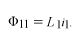(2)

The units of inductance are henrys. If a second circuit is present, some of the field B1 will pass through circuit 2 and there will be a magnetic flux Φ21in circuit 2 due to the current i1. The mutual inductance M21 is given by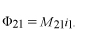(3)

The magnetic flux in circuit 1 due to a current in circuit 2 is given by Φ12 = M12i2. An important property of the mutual inductance is that M21 = M12. It is therefore sufficient to use the label M without subscripts for the mutual inductance of two circuits.
The value of the mutual inductance of two circuits can range from +Square root ofL1L2to −Square root ofL1L2, depending on the flux linkage between the circuits. If the two circuits are very far apart or if the field of one circuit provides no magnetic flux through the other circuit, the mutual inductance is zero. The maximum possible value of the mutual inductance of two circuits is approached as the two circuits produce B fields with increasingly similar spatial configurations.
If the rate of change with respect to time is taken for the terms on both sides of equation, the result is dΦ11/dt = L1di1/dt. According to Faraday’s law, dΦ11/dt is the negative of the induced electromotive force. The result is the equation frequently used for a single inductor in an AC circuit—i.e.,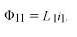(2)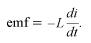(4)

The phenomenon of self-induction was first recognized by the American scientist Joseph Henry. He was able to generate large and spectacular electric arcs by interrupting the current in a large copper coil with many turns. While a steady current is flowing in a coil, the energy in the magnetic field is given by 1/2 Li2. If both the inductance L and the current i are large, the amount of energy is also large. If the current is interrupted, as, for example, by opening a knife-blade switch, the current and therefore the magnetic flux through the coil drop quickly. Equation describes the resulting electromotive force induced in the coil, and a large potential difference is developed between the two poles of the switch. The energy stored in the magnetic field of the coil is dissipated as heat and radiation in an electric arc across the space between the terminals of the switch. Due to advances in superconducting wires for electromagnets, it is possible to use large magnets with magnetic fields of several teslas for temporarily storing electric energy as energy in the magnetic field. This is done to accommodate short-term fluctuations in the consumption of electric power.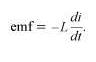(4)

transformer is an example of a device that uses circuits with maximum mutual induction.Figure 5 illustrates the configuration of a typical transformer. Here, coils of insulated conducting wire are wound around a ring of iron constructed of thin isolated laminations or sheets. The laminations minimize eddy currents in the iron. Eddy currents are circulatory currents induced in the metal by the changing magnetic field. These currents produce an undesirable by-product—heat in the iron. Energy loss in a transformer can be reduced by using thinner laminations, very “soft” (low-carbon) iron and wire with a larger cross section, or by winding the primary and secondary circuits with conductors that have very low resistance. Unfortunately, reducing the heat loss increases the cost of transformers. Transformers used to transmit and distribute power are commonly 98 to 99 percent efficient. While eddy currents are a problem in transformers, they are useful for heating objects in a vacuum. Eddy currents are induced in the object to be heated by surrounding a relatively nonconducting vacuum enclosure with a coil carrying a high-frequency alternating current.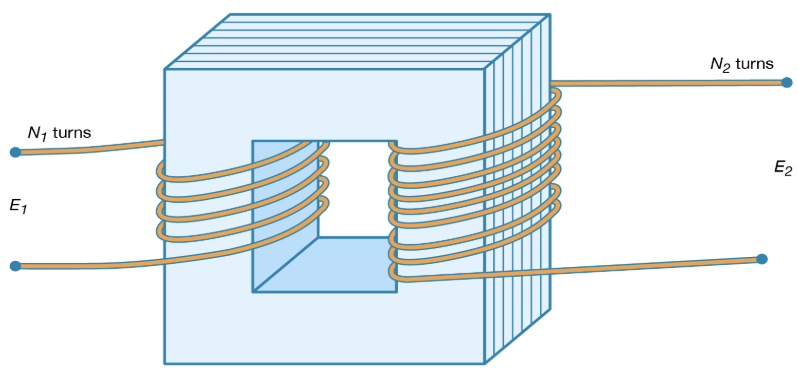Figure 5: An AC transformer (see text).

In a transformer, the iron ensures that nearly all the lines of B passing through one circuit also pass through the second circuit and that, in fact, essentially all the magnetic flux is confined to the iron. Each turn of the conducting coils has the same magnetic flux; thus, the total flux for each coil is proportional to the number of turns in the coil. As a result, if a source of sinusoidally varying electromotive force is connected to one coil, the electromotive force in the second coil is given by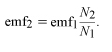(5)

Thus, depending on the ratio of N2 to N1 (where N1 and N2 are the number of turns in the first and second coils, respectively), the transformer can be either a step-up or a step-down device for alternating voltages. For many reasons, including safety, generation and consumption of electric power occur at relatively low voltages. Step-up transformers are used to obtain high voltages before electric power is transmitted, since for a given amount of power, the current in the transmission lines is much smaller. This minimizes energy lost by resistive heating of the conductors.
Faraday’s law constitutes the basis for the power industry and for the transformation of mechanical energy into electric energy. In 1821, a decade before his discovery of magnetic induction, Faraday conducted experiments with electric wires rotating around compass needles. This earlier work, in which a wire carrying a current rotated around a magnetized needle and a magnetic needle was made to rotate around a wire carrying an electric current, provided the groundwork for the development of the electric motor.

Offer running on EduRev: Apply code STAYHOME200 to get INR 200 off on our premium plan EduRev Infinity!

## Basic Physics for IIT JAM

77 videos|156 docs|94 tests

,

,

,

,

,

,

,

,

,

,

,

,

,

,

,

,

,

,

,

,

,

;